﻿ The Immersive Virtual Reality: A Study in Three-dimensional Euclidean SpacePublications are Open
Access in this journal
Article Versions
Export Article
• Normal Style
• MLA Style
• APA Style
• Chicago Style
Research Article
Open Access Peer-reviewed

### The Immersive Virtual Reality: A Study in Three-dimensional Euclidean Space

Karla Liliana Puga Nathal, María Eugenia Puga Nathal, Humberto Bracamontes del Toro , Marco Antonio Guzmán Solano, Juan Carlos Martínez Sandoval
American Journal of Educational Research. 2018, 6(3), 170-174. DOI: 10.12691/education-6-3-2
Published online: March 08, 2018

### Abstract

New technologies, since the eighties have dabbled in classroom education as a support tool in teaching activities. The learning problems in the discipline of mathematics faced by students at different educational levels are known. However, various investigations have shown favorable results in the appropriation of concepts if the methodology of work in the classroom is supported by means and materials that support the student to learn the mathematical contents, even autonomously, in an alternative learning environment [1,2]. This paper shows the results of a research whose objective was to generate and evaluate an instructional scenario for the learning of vector themes and three-dimensional space. Students, from the manipulation of physical and virtual objects (mediated by recognition of patterns and mixed reality), interact with mathematical concepts essential to understand the geometry of three-dimensional space and the interpretation of various figures such as solids and cylinders. This interaction promotes the extraction of the data necessary for the mathematical modeling of a contextualized phenomenon. For that, it was necessary to investigate on the one hand, the conceptual elements that take place in the student's processes in the appropriation of mathematical concepts and on the other, the effects that the instructional design produces based on the manipulation of physical and virtual objects on the learning of the mentioned mathematical concepts. The research shows, first of all, the feasibility of introducing virtual scenarios for the manipulation of mathematical objects in the classroom as well as the promotion of the development of didactic materials mediated by new technologies for the learning of mathematics. Core point for strengthening the construction of mathematical knowledge of students.

### 1. Introduction

According to the competency-based educational model 3, at present, the mathematical training of an individual within the classroom must take place not only as the acquisition of a set of rules, procedures and knowledge; if not that it must provide the individual with the necessary elements to develop their ability to analyze, reason and communicate mathematical ideas in an effective way to pose, formulate and solve mathematical problems in different situations, whether in a personal, educational, professional, social or scientific. This is having developed mathematical competence 4.

However, the staging of mathematical skills, requires the student -in the cognitive aspect- a level of understanding of the central concept that is immersed in a problem situation. For example, the calculation of the volume of the figure that is generated when intersecting two PVC pipes of diameter a. It is expected that the student activates in his mind the concept of multiple integral to be able to give solution to this situation. If the student does not understand the mathematical concept immersed, or if the level of understanding of it is not enough, this will represent an obstacle to respond to this approach.

In this regard, the question arises: How to promote the appropriation of mathematical concepts? What are the effects produced by didactic strategies based on the manipulation of physical and virtual objects in the appropriation and application of mathematical concepts? The search for answers to these questions leads to the need to generate learning environments where students can put their knowledge and mathematical skills into practice.

In the present investigation a scenario was created, where, from the manipulation of physical objects and through new technologies, two things were promoted: first, the understanding of mathematical concepts and then, the solution of problem situations that involve interpreting, modeling, taking correct decisions, discerning and explaining the mathematical behavior of various useful phenomena for the training of all students of engineering careers.

### 2. Background

The vector calculation is a subject that is located in the first semesters of the engineering careers that are offered at the National Technological Institute of Mexico (TNM). In it, students are introduced to the study of vectors, figures and surfaces in three-dimensional space. The importance of these contents in the basic grid is fundamental because they address core concepts for the subsequent courses of mathematics and other areas of knowledge, for example, in the field of physics.

When the engineering student is introduced for the first time to the study of mathematical objects represented in R3, such as points, vectors, lines, planes, quadratic surfaces, cylinders, etc., it has been observed that it presents difficulties in its geometric interpretation and its relation to its algebraic record. In part, this is because students must not only geometrically represent those objects on a flat surface (notebook) where they simulate the structure of three-dimensional space, but they lack skills in drawing figures and in most cases they must extract relevant information from such traces.

It is documented that the construction of a mathematical concept is not an immediate activity, it requires different stages that can be developed in variable periods of time and also require work and dedication of the individual 1. These stages involve the processes and construction of objects. The processes are understood, as the stage in which a series of operations can be developed and coordinated in the student's mind "the student will then have acquired an operational thinking with respect to that concept ..." 1. When the process is internalized and materialized in the mind of a student, a unique entity called object is generated. Once the object has been acquired the individual has developed some ability to think of this concept either dynamically as a process, or static as an object.

On the other hand, this research is based on the premise that concepts in mathematics can be treated from different registers or frames of representation: numerical, algebraic, analytical, contextual and visual 5. The latter includes geometric aspects such as graphs, diagrams and drawings 6.

For this reason, there is a need to generate learning scenarios where students, aided by new technologies, can manipulate, analyze and interpret the main elements that characterize mathematical objects. All this with the purpose of promoting the appropriation of concepts and the links between their diverse registers of representation, mainly between the algebraic and the geometric, the latter being essential for the generation of mathematical models that allow to solve contextualized situations.

Currently, virtual reality is increasingly incorporated into educational settings, but there is still little scientific production about its impact in the classroom, mainly in engineering mathematics. However, there are some approaches to this technology aimed at teaching.

Several investigations 7, address the problem regarding the performance of graduates of the electrical engineering career. In this work, the creation of a virtual world is promoted in which electrical systems can be analyzed, with which more institutions can carry out corresponding studies, reducing risks and costs that are not counted in the laboratories 8.

There are investigations 9 in which the Leap Motion was incorporated to manipulate three-dimensional objects. A study was conducted to evaluate its impact on fourth grade students at the time of defining the concepts of faces, edges and vertices which resulted in students achieving a better understanding of mathematical objects. However, in this proposal only forms were manipulated, no algebraic representation records were involved, which are essential in a mathematical modeling.

In other investigation 10, propose an application with augmented reality that shows three-dimensional objects called augmented geometrical bodies to observe the interest generated by using this type of technology in teaching in basic study. However, the study did not address the manipulation of objects and their relationship with mathematical models, only the information of three-dimensional objects was shown. In this work the physical form of the three-dimensional objects is modified and manipulated and the repercussions that this entails in the corresponding mathematical model are observed.

In 11 proposes a curriculum that shows students' learning within a virtual reality immersed in comparison with learning in a common classroom. To do this, a virtual world that can be used freely known as Second Life is used to make sections of classes within it. Improvements in student learning were observed compared to students who attended face-to-face classes (without access to Second Life). However, the didactic intention of this scenario is more towards a socialization of learning, a more everyday mathematics, it does not focus on a mathematics applied to engineering, where an analysis of three-dimensional figures is promoted.

### 3. Contextualization

Vectors and three-dimensional space are basic concepts in the training of every engineer since they solve problems in specific contexts that could not be explained by other mathematical concepts. Contexts that yield mathematical models that involve two or more variables.

For example, in physics courses, the vector concept is necessary to be able to describe mathematically the space in which we live, vectors such as force, speed and acceleration are related to living space. Vectors are fundamental in kinematics, in dynamics, in electricity or in the study of the behavior of magnetic fields. On the other hand, the study of three-dimensional space enables the student to incorporate more detailed mathematical models, a deeper analysis, as well as correct and well-founded decisions about a phenomenon specific to their engineering in which several variables intervene.

The application of vectors is extensive. In engineering schools, the concept of vector is approached to model and analyze various situations, for example in vector calculation, static of the particle and the rigid body, particle and rigid body dynamics, mechanical vibrations, thermodynamics and electricity and magnetism. In most of these cases, the basic idea of projecting a force F in a reference system is used. For this reason, the geometric representation becomes indispensable and the student must relate a series of concepts that involve the idea of vector, such as magnitude, direction, projections of vectors, vector products, etc.

When the force is represented in a two-dimensional reference system, it has been observed that the students have no greater difficulty in defining the forces that generate F, and in this way arrive at expressions such as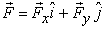. However, the scenario becomes different when the force is represented in three-dimensional space. It has been observed that students lack elements that allow them, at first, to visualize a point in R3, identify the direction of the force, as well as project it on the axes of coordinates to define its components.

Points and vectors in three-dimensional space are a topic of discussion for the construction of mathematical concepts. However, there are other concepts that must be treated in 3D such as multiple integrals, straight lines, planes, solids and cylinders, essential in the modeling of mathematical situations and for the construction of other concepts. If students have difficulties in interpreting vectors and points in third dimension, it is chaotic for them to build and interpret graphs and even more to find the intersections between them and solve the applications that this refers to.

### 4. Fundamentals of the Proposal

The epistemological foundation of the research is based, on the one hand, from the Theory of Semiotic Representations 12. It is established that mathematical concepts, unlike other concepts are treated from different registers of representation, since they are those that allow access and application to mathematical objects. Part of the theory of Duval 12 raises as an object of study an analysis of the cognitive processes that takes place when an individual move from one record of representation to another of the same concept.

A core element in the research was the concept of Immersive Virtual Reality. This term is used to refer to a scenario that simulates the sensory perceptions of the user. He uses interface devices pretending to make him feel that he is inside the world generated by the computer, which is part of the scenario and not only as a spectator, as it happens in the majority of cases in which virtual reality is used 13.

In the research, virtual platforms were designed and developed in which the user related mathematical objects such as graphs in R3 and their equations. The objects are manipulated by means of corporal gestures to establish relationships and promote the transit between registers of geometric representation and algebraic registers.

The mediators between the scenario and the mathematical concepts were the following:

a) An application set in Augmented Reality, where students can visualize the graphs of the quadratic surfaces from different visual perspectives, can be seen in Figure 1.

b) An application set in Virtual Reality that deals with a museum, where the user can access a gallery containing quadratic surfaces and cylinders as shown in Figure 2.

• Figure 1. Quadratic surface and its traces in an augmented reality environment
• Figure 2. Quadratic surfaces and cylinders in a virtual reality environment

c) An application set in an immersive virtual reality scenario, mediated by the Leap Motion controller, where the user on his computer can interact with geometric objects such as vectors and points in three-dimensional space. The user, through movements with his hands, can modify the physical conditions of the mathematical objects that appear on his screen and this causes a change in the mathematical model of said object. Figure 3.

• Figure 3. Three-dimensional Euclidean space in an immersive virtual reality environment

d) An application established in immersive virtual reality 13, mediated by the Kinect controller, where users, through body movements, modify the physical characteristics of mathematical objects such as quadratic surfaces and cylinders and this leads to a variation of the descriptive parameters in their mathematical model. Figure 4.

e) 3D impressions of mathematical objects, where students manipulate physical objects and share their geometric characteristics generating their mathematical model. Figure 5.

• Figure 4. Quadratic surfaces in an immersive virtual reality environment
• Figure 5. 3D printing of quadratic surfaces and cylinders

On the other hand, the development of didactic instruments was supported by the user-centered design approach 14, which has as its central axis the creation of products that meet the specific needs of the user. This approach proposes the following stages in the design of a product (taken verbatim from 14):

Planning / Research: The product is defined conceptually based on the research of the objective audience (needs, motivations, characteristics, habits, mental model, activities ...) and competitive analysis (which other products exist with similar audiences and functions).

Design / Prototyping: Design decisions are made starting from its most general dimension (information architecture and interaction design) up to its most specific dimension (detailed graphic design and micro-interactions). These decisions are documented and prototyped with evaluation objectives.

Evaluation: Those design decisions and critical product processes are tested through evaluation methods that can involve users.

Implementation: Once the design has reached the required level of quality, it is then implemented or put into production.

Monitoring: Once the product is launched, it is studied how users use it, in order to identify opportunities for improvement.

The research considered as a starting point the manipulation of physical and virtual objects as mediators to promote the appropriation of concepts involved with the three-dimensional space and the geometry that this implies. Through such objects students will be able to verify and understand the concepts used within scenarios that include the three-dimensional Euclidean space. In such scenarios, concepts are presented from their register of geometric representation and from their algebraic representation (Figure 6).

• Figure 6. Relationship between two representation records

At first, the concepts were treated from their register of algebraic representation, and through the use of GeoGebra software. Relationships were established between said register and the graph of each cylinder and surface.

Subsequently, the students, through a viewer and the Museum VR ITCG application (Figure 2, was published in PlayStore), are introduced to a virtual world, where the figures take shape in their natural setting and can be observed and analyzed from different perspectives. For the study of the traces of each surface, a tablet and smartphone was used where students could appreciate them from an augmented reality environment through the CVectorial ITCG application (Figure 1, published in PlayStore).

One of the intentions of the research was to observe how students establish relationships between geometric and algebraic representation registers. For this, it was necessary first to introduce them in a virtual world where they could modify geometric conditions and describe the effect that this entails in their mathematical model. To do this, the applications developed for the immersion to the virtual scene using the Leap Motion (Figure 3) and Kinect (Figure 4) controls were used.

To give an account of the students' achievements, various cylinders and quadratic surfaces were printed in 3D, which were provided with the intention of identifying their name and estimating a mathematical model that best describes their physical form. The students had to record in writing all their processes and findings.

### 5. Methodology

The research was developed in two stages at the facilities of the Technological Institute of Cd. Guzmán. Firstly, the instructional design was implemented, which consisted of a series of systematically planned activities that were carried out by the students involved in the research. It includes the design and evaluation of the virtual environment and each of the applications for each scenario (this seen from an epistemological perspective and according to the user-centered design approach), a working notebook and three-dimensional printed objects. In this stage it was necessary to create a multidisciplinary team composed of developers who were responsible for the design and development of the virtual environment and teachers of mathematics, who were responsible for the didactic part and methodology of the proposal.

A second moment of the investigation consisted of the staging of the proposal to account for the effects of this on the students own learning, with respect to the mathematical concepts addressed. A design of type of quasi-experimental was implemented, a comparative study was made between the means of two groups of third semester of ITCG computer systems engineering career. The groups were called experimental group and control group.

A t-student statistical analysis with a level of significance of 95% (one tail) was performed to evaluate if the mean (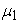) of the experimental group is significantly higher than the mean (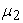) of the control group.

Symbolically, the hypotheses are established as:

Null hypothesis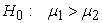Alternative hypothesis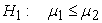whereandare the means of the samples, control and experimental respectively.

The evaluation of the proposal was made by comparing the value of the test statistic, t obtained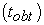and the critical t value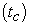that depends on the degrees of freedom and the level of significance. The acceptance rule of the null hypothesis was established as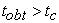. The results obtained were that the mean of the experimental group was significantly higher than those of the control group.

A qualitative study was carried out in which the relevance of the proposal as well as the development of its contents were assessed. For this, in-depth interviews 15, the technique of interviewing and observation were carried out and in this way to inquire about the processes that take place in the mind of a student when manipulating physical and virtual objects. In this way the design of such materials is strengthened.

### 6. Results and Conclusions

Statistically the study yielded favorable results, since the students that participated showed abilities to relate quadratic surfaces located in different ways in the three-dimensional Euclidean space with their mathematical model, they managed to establish relations between models and graphs bi-directionally and characterize each mathematical object that was presented to them.

The didactic proposal not only promoted skills and learning in the students who participated in the experiment but it was a motivating element, they worked with enthusiasm, with a disposition for how dynamic and attractive the materials were. The work in the classroom that required the proposal was 4 hours and approximately 8 hours outside the classroom, according to the students' reports they spent more time extra-class than estimated because they decided to spend more time in the virtual stages.

The combination of the virtual stage and the printing of 3D figures was convenient in the study because the students observed in their natural environment the 3D objects, manipulated them and managed to obtain data from them for the construction of mathematical models.

One of the valuable contributions of the research is the inclusion of immersive virtual scenarios in the classroom, since this opens up an ocean of possibilities in the didactic structure of different courses. It can be applied not only in mathematics but also in other spheres of science as in electronics, electrical, mechanics, architecture, environment, etc, Due to the possibility offered by these virtual environments for the simulation of reality, virtual laboratories could be implemented where students can build diverse learning through the manipulation of objects.

### References

Published with license by Science and Education Publishing, Copyright © 2018 Karla Liliana Puga Nathal, María Eugenia Puga Nathal, Humberto Bracamontes del Toro, Marco Antonio Guzmán Solano and Juan Carlos Martínez SandovalThis work is licensed under a Creative Commons Attribution 4.0 International License. To view a copy of this license, visit http://creativecommons.org/licenses/by/4.0/